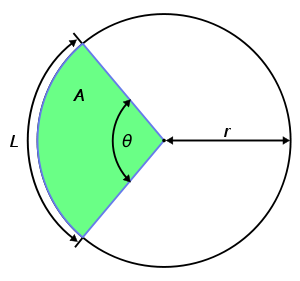# Circular sector

﻿
Circular sectorA circular sector is shaded in green

A circular sector or circle sector, is the portion of a disk enclosed by two radii and an arc, where the smaller area is known as the minor sector and the larger being the major sector. In the diagram, θ is the central angle in radians, r the radius of the circle, and L is the arc length of the minor sector.

A sector with the central angle of 180° is called a semicircle. Sectors with other central angles are sometimes given special names, these include quadrants (90°), sextants (60°) and octants (45°).

The angle formed by connecting the endpoints of the arc to any point on the circumference that is not in the sector is equal to half the central angle.

## Area

The total area of a circle is πr2. The area of the sector can be obtained by multiplying the circle's area by the ratio of the angle and (because the area of the sector is proportional to the angle, and is the angle for the whole circle):$A = \pi r^2 \cdot \frac{\theta}{2 \pi} = \frac{r^2 \theta}{2}$

Another approach is to consider this area as the result of the following integral :$A = \int_0^\theta\int_0^r dS=\int_0^\theta\int_0^r \tilde{r} d\tilde{r} d\tilde{\theta} = \int_0^\theta \frac{1}{2} r^2 d\tilde{\theta} = \frac{r^2 \theta}{2}$

Converting the central angle into degrees gives$A = \pi r^2 \cdot \frac{\theta ^{\circ}}{360}$

## Perimeter

The length of the perimeter of a sector is the sum of the arc length and the two radii:$P = L + 2r = \theta r + 2r = r \left( \theta + 2 \right)$

## Center of Mass

The distance from the center of the circle (that the sector is a part of) to the center of mass of the sector is two thirds of the corresponding distance for the center of mass of the arc of the sector. In particular, as the central angle approaches zero the center of mass of the arc is at distance r from the center of the circle, so that of the sector is at distance 2r/3. As the central angle approaches 2π (the whole circle), the center of mass of the arc converges to the center of the circle, whence so does that of the circular sector.

Wikimedia Foundation. 2010.

### Look at other dictionaries:

• circular sector — noun the portion of a circle enclosed by two radii and an arc …   Wiktionary

• Circular — is a basic geometric shape such as a Circle. Contents 1 Documents 2 Travel and transportation 3 Places …   Wikipedia

• Sector — may refer to: * Sector, Devon, a location in the county of Devon in south western England * Sector, West Virginia, an unincorporated community in Hampshire County, West Virginia, United States of America * Sector (economic): one of several… …   Wikipedia

• Circular segment — In geometry, a circular segment is an area of a circle informally defined as an area which is cut off from the rest of the circle by a secant or a chord. The circle segment constitutes the part between the secant and an arc, excluding the circle… …   Wikipedia

• Sector circular — de ángulo θ. Se denomina sector circular a la porción de círculo comprendida entre un arco de circunferencia y sus respectivos radios delimitadores. Área El área de un sector circular depende de dos parámetros, el radio y el ángulo central, y… …   Wikipedia Español

• Circular — puede referirse a: Relativo al círculo. Documento preparado para comunicar un mensaje idéntico a un grupo (círculo) de personas o al público en general. En geometría y otras ramas de matemática: Sector circular. Segmento circular. Corona circular …   Wikipedia Español

• Circular flow of income — In this simplified image, the relationship between the decision makers in the circular flow model is shown. Larger arrows show primary factors, whilst the red smaller arrows show subsequent or secondary factors. In economics, the terms circular… …   Wikipedia

• sector — (Del lat. sector.) ► sustantivo masculino 1 ADMINISTRACIÓN Parte de una ciudad, local grande u otro lugar: ■ hay un repartidor de paquetes para cada sector de la ciudad. 2 Parte de una clase o de una colectividad que presenta caracteres… …   Enciclopedia Universal

• Sector — El término sector hace referencia a la parte seccionada o cortada de un todo. Desde su origen etimológico se extiende a muchos otros términos por analogía: Checkpoint Charlie: Está usted saliendo del sector americano. Contenido …   Wikipedia Español

• Sector instrument — A five sector mass spectrometer A sector instrument is a general term for a class of mass spectrometer that uses a static electric or magnetic sector or some combination of the two (separately in space) as a mass analyzer. A popular… …   Wikipedia